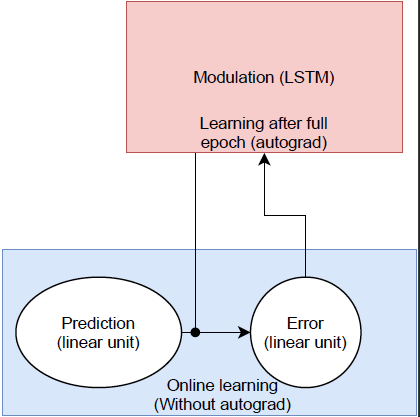# Learning rate modulation and gradient descent

Hello !

I was trying to implement a model these last few days and I stumbled across problem that I can not solve:
Here is the newtork (simplified)The blue part has its `requires_grad` set to False, and I manually update the weight as:

`self.pred.weight.data = self.pred.weight.data + 0.1 * modulation * deltaW[0,0].T`
and
`modulation = torch.mean(out_modulation)`.

To make it clear I run a simulation of N steps and every time step I update the weights manually of the online layer.
After all these steps I want to minimize the overall error by doing a backprop (autograd) over the red area. where the error is the sum of the prediction error in blue area (i.e. the error does not explicitly depend on the modulation population).

The problem is since the error does not depend explicilty of the red area, pytorch can not estimate a gradient. Is there a way to make the graph understand that the manual updates is dependent of the modulation population ?

full forward code:

` def forward(self, previous, observation,hidden_modulation):

``````    prediction = self.pred(previous)  #linear unit
prediction_error = observation - prediction

out_modulation, hidden_modulation = self.surprise(torch.abs(prediction_error), hidden_modulation) #LSTM
modulation = torch.mean(torch.sigmoid(out_modulation))
deltaW = (
torch.einsum(
"bij,bik->bijk",
previous,
prediction_error))
self.pred.weight.data = self.pred.weight.data + modulation * deltaW[0,0].T #online update
self.pred.weight.data[self.pred.weight.data < 0] = 0
return prediction_error, hidden_pred, hidden_surprise,torch.relu(prediction),modulation```````

What happens when you don’t use `.data` here?

thanks for you reply. without data I can not assign a tensor

`cannot assign 'torch.FloatTensor' as parameter 'weight' (torch.nn.Parameter or None expected)`